# NCERT Example 26 Chapter 5 Class 12 Maths Find the derivative of f given by

September 14, 2022## Description

Example 26 Find the derivative of f given by { \displaystyle f(x) = \sin^{–1} x } assuming it exists.07:01

## If x sin t and y sin pt prove that

If {\displaystyle x = \sin t} and {\displaystyle y = \sin pt } , prove that {\displaystyle (1 - x^2)\frac{d^{2}y}{dx^2} - x\frac{dy}{dx} + p^2y = 0 } .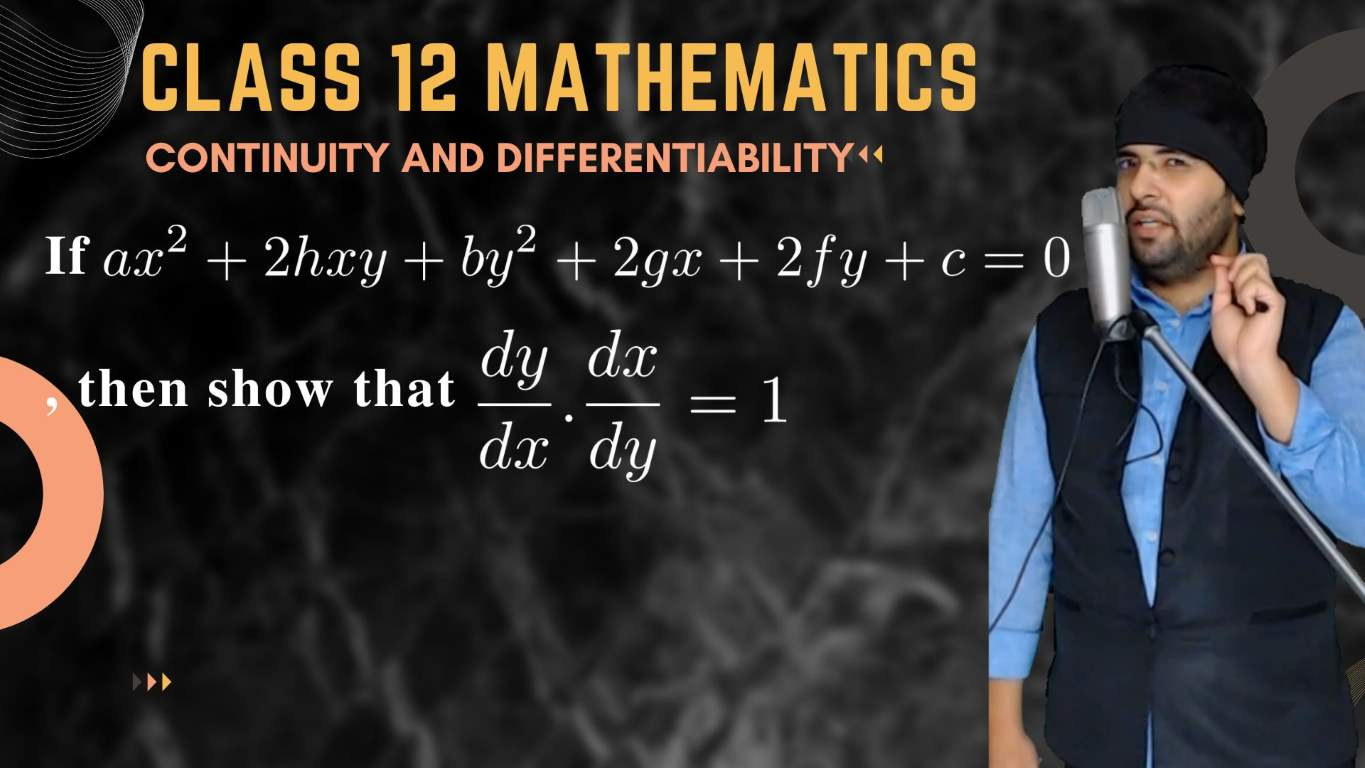07:36

## If then show that dy over dx times dx over dy equals 1

If {\displaystyle a x^2 + 2hxy + b y^2 + 2gx + 2fy + c = 0 } , then show that {\displaystyle \frac{dy}{dx} . \frac{dx}{dy} = 1 } .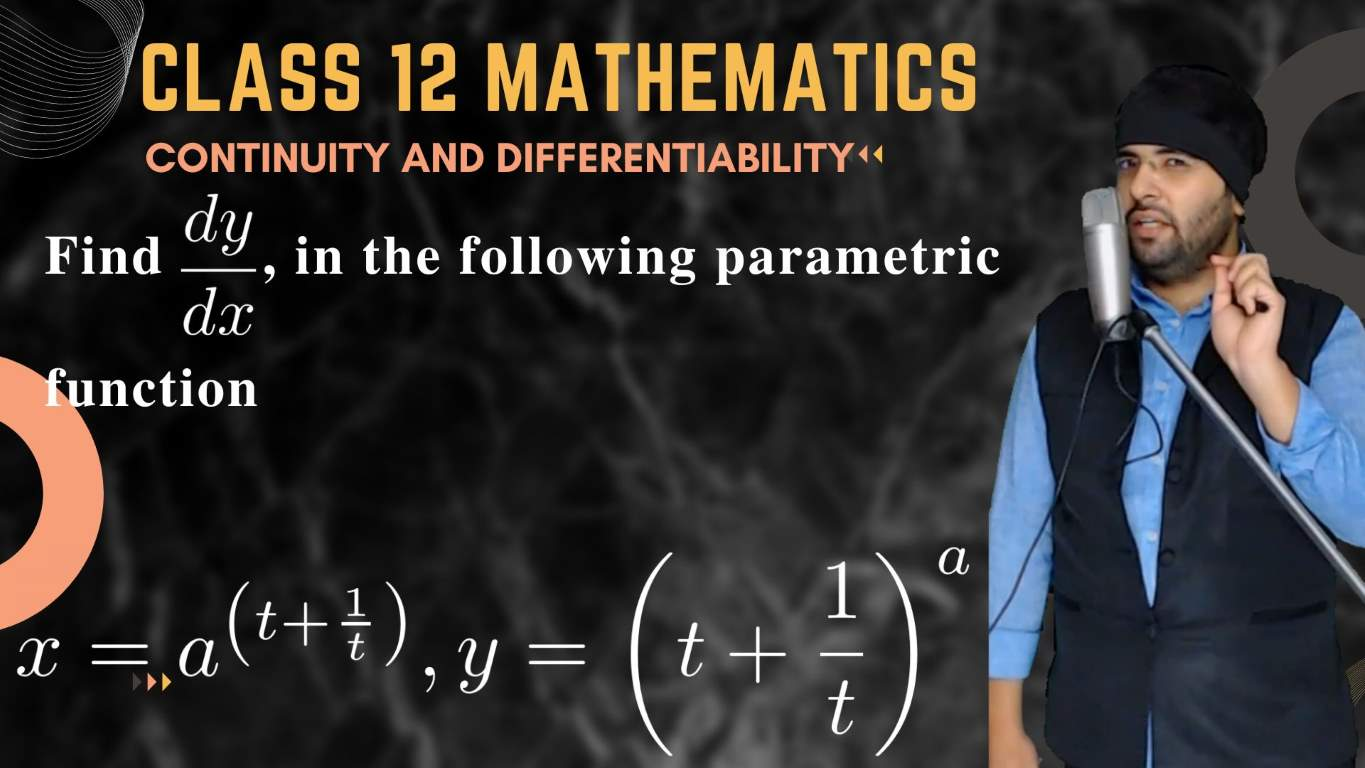04:31

## NCERT Example – 47 Continuity and Differentiability Chapter 5

NCERT Example – 47 Continuity and Differentiability Chapter 5: Find {\displaystyle \frac{dy}{dx} } in the following parametric function {\displaystyle x = a^{\left ( t+\frac{1}{t} \right )}, y = {\left ( t+\frac{1}{t} \right )}^a} .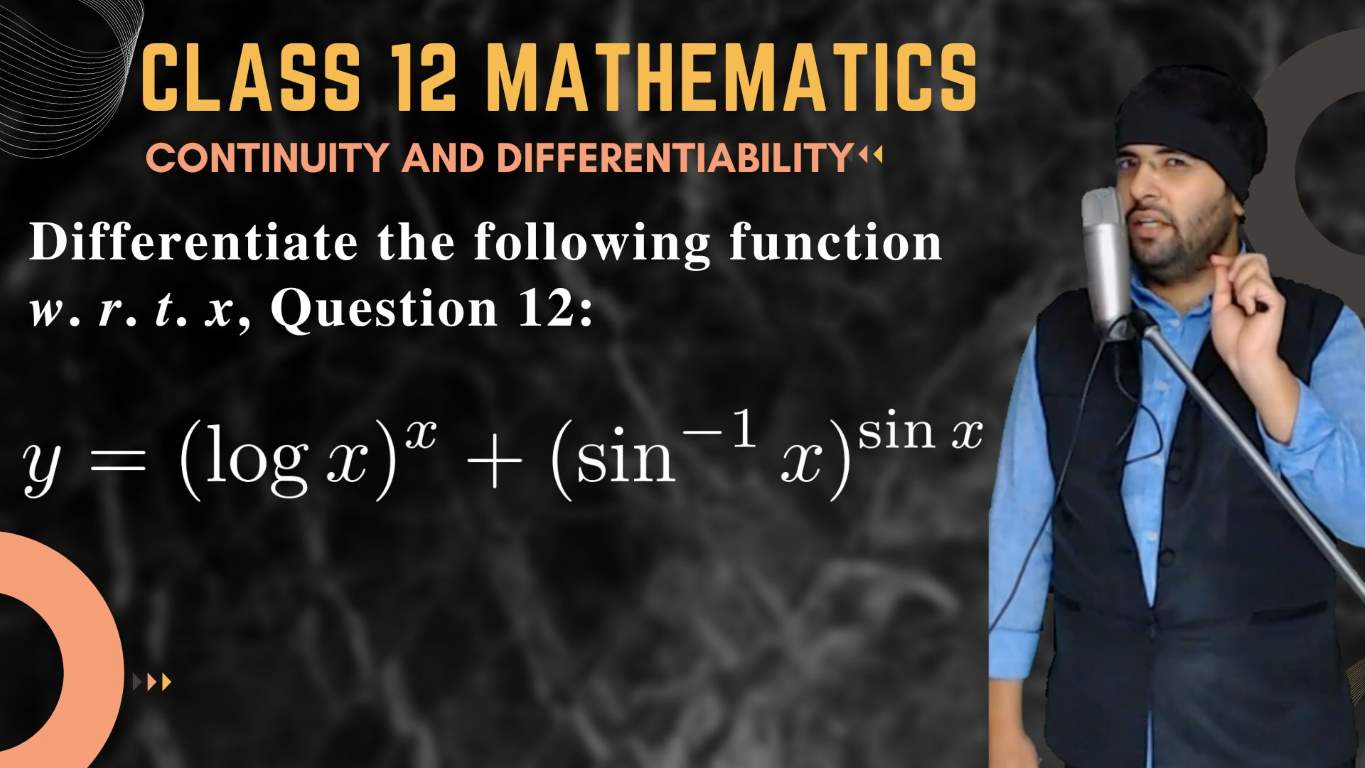07:23

## y = (log x)^x + (sin^(-1)x)^(sin x)

Differentiate the following function w. r. t. x, Question 12: {\displaystyle y = (\log x)^x + (\sin^{-1}x)^{\sin x} }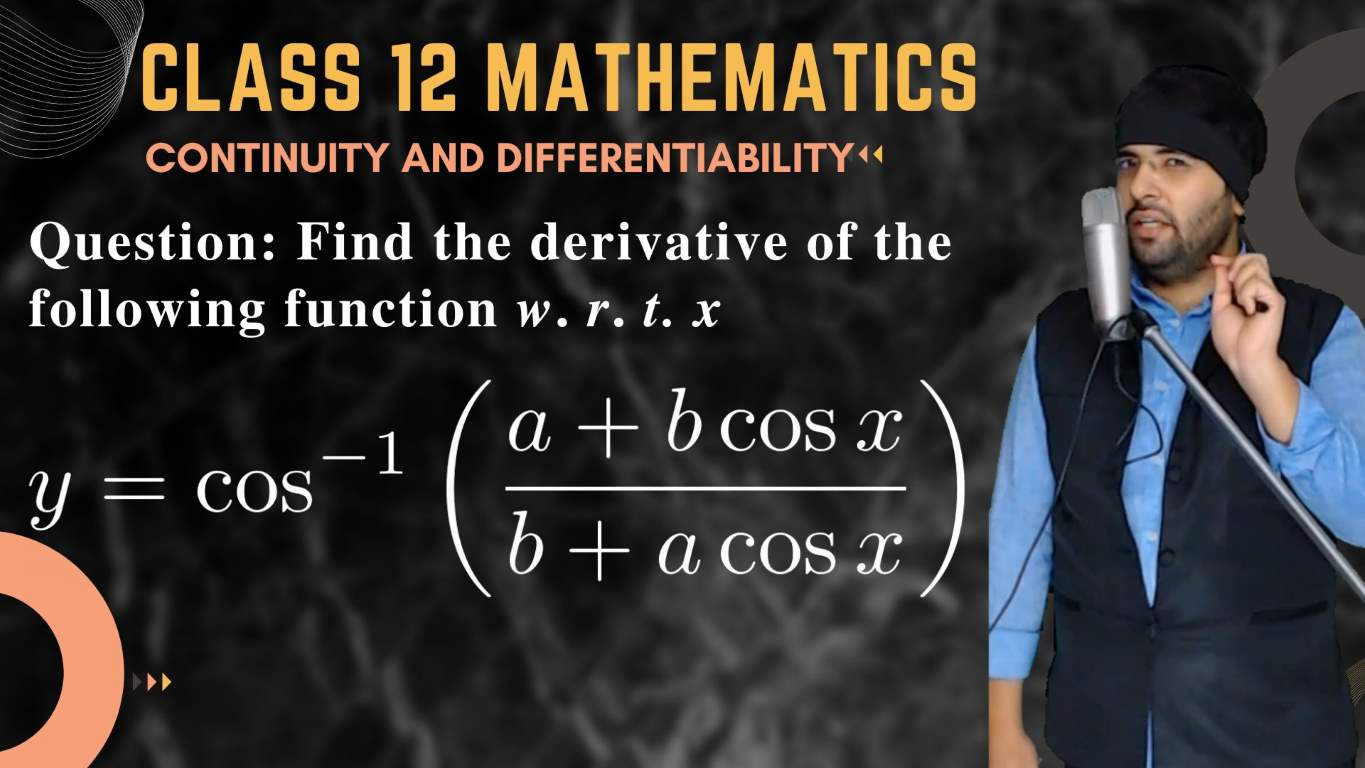10:07

## Find the derivative of the following function w.r.t x

Question: Find the derivative of the following function w.r.t x, {\displaystyle y = \cos^{-1}\left( \frac{a + b\cos x}{b + a \cos x} \right) }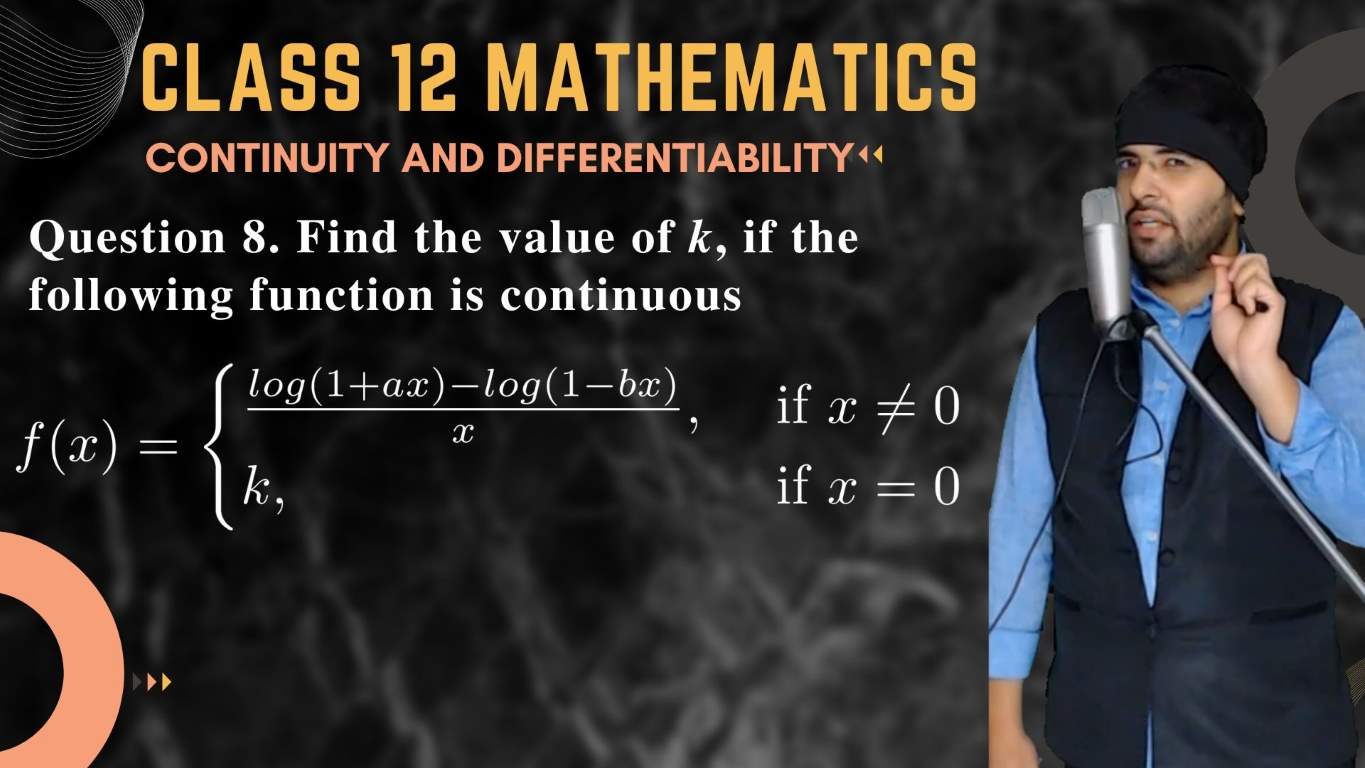05:39

## Find the value of k, if the following function is continuous

Question 8. Find the value of k, if the following function is continuous {\displaystyle f(x)=\begin{cases} \frac{log (1+ax)-log (1-bx)}{x}, & \text{ if } x\ne 0 \\ k, & \text{ if } x=0 \end{cases} }08:19

## If (x - a)^2 + (y - b)^2 = c^2 is a constant independent of a and b.

Question 15. If { \displaystyle (x - a)^2 + (y - b)^2 = c^2 } , for some c > 0, prove that {\displaystyle \frac{\left[ 1 + \left( \frac{dy}{dx}\right )^2 \right ]^{\frac{3}{2}}}{\frac{d^2y}{dx^2}} } is a constant independent of a and b.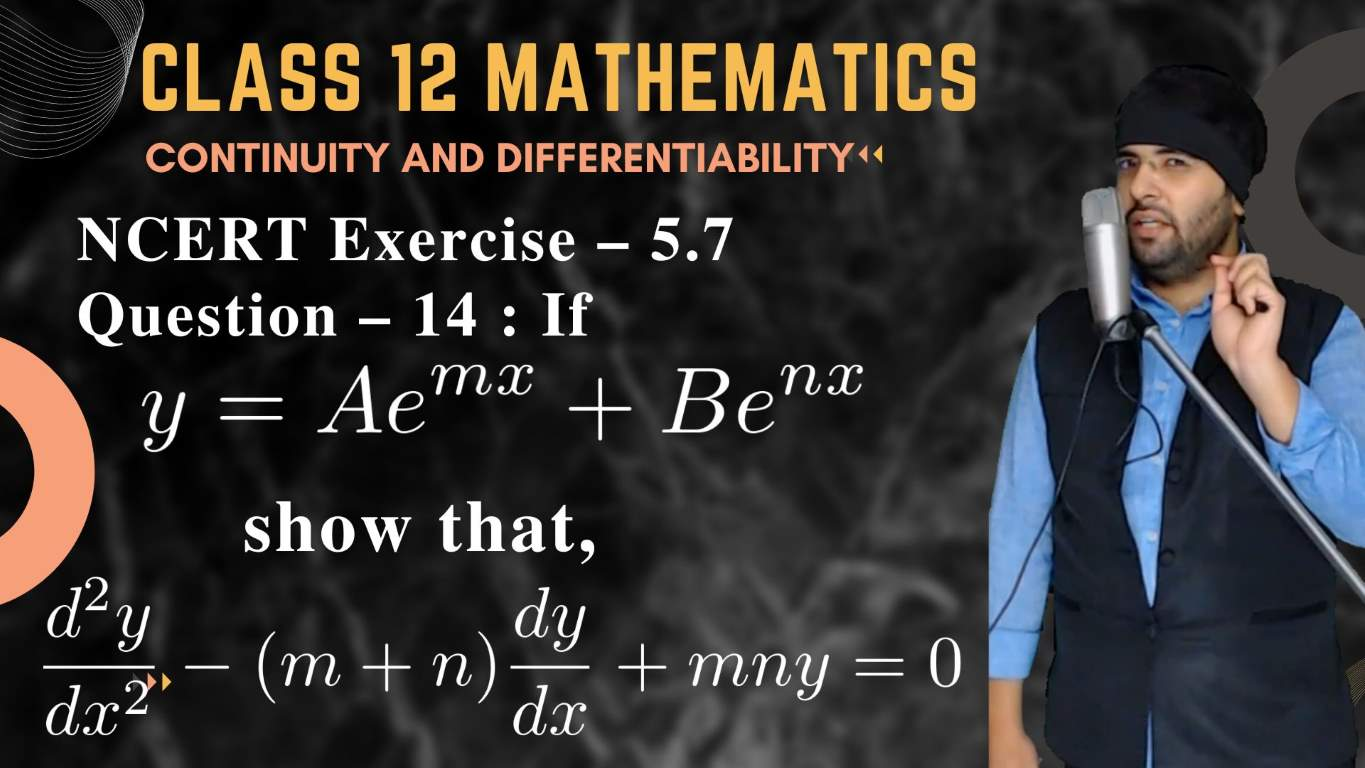04:39

## Continuity and Differentiability Class 12 Maths NCERT Exercise – 5.7 Question – 14

Question 14. If { \displaystyle y = Ae^{mx} + Be^{nx}} , show that { \displaystyle \frac{d^2{y}}{dx^2} - (m + n)\frac{dy}{dx} + mny = 0 } .17:42

## Ch05. Continuity and Differentiability Class 12 Maths NCERT Exercise – 5.6 Question – 7

Question 7 : { \displaystyle x = \frac{\sin^3{t}}{\sqrt{\cos 2t}},  y = \frac{\cos^3{t}}{\sqrt{\cos 2t}} }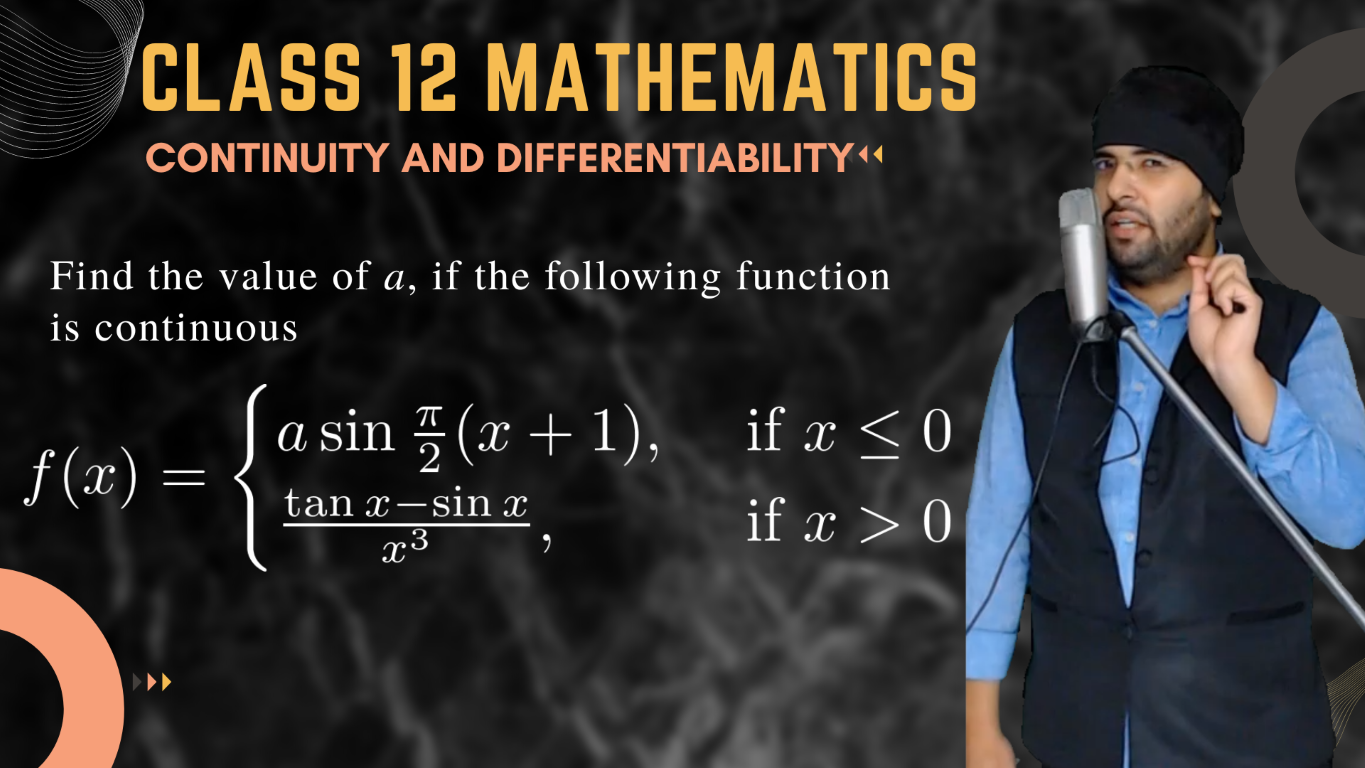05:21

## Find the value of a, if the following function is continuous

Find the value of a, if the following function is continuous
Question 17: {\displaystyle f(x) = \begin{cases} a \sin \frac{\pi }{2} (x+1), & \text{ if } x \le 0 \\ \frac{\tan x-\sin x}{x^{3} }, & \text{ if } x > 0 \end{cases}}

M A T H Y U G